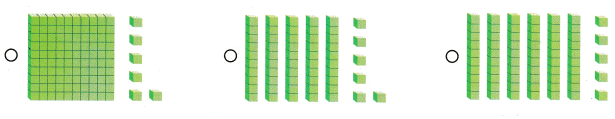# Texas Go Math Grade 1 Lesson 1.5 Answer Key Tens and Ones to 120

Refer to our Texas Go Math Grade 1 Answer Key Pdf to score good marks in the exams. Test yourself by practicing the problems from Texas Go Math Grade 1 Lesson 1.5 Answer Key Tens and Ones to 120.

## Texas Go Math Grade 1 Lesson 1.5 Answer Key Tens and Ones to 120

Essential Question
How can you show numbers to 120 as hundreds, tens, and ones?
Explanation:
1 box = 100
1 line = 10
1 dot = 1
1 box 2 lines and 0 dots = 120

Explore
Use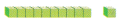to model the number.
Draw a quick picture to show your work.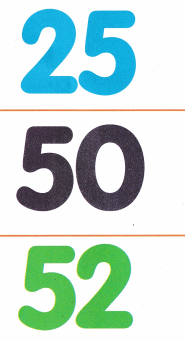For The Teacher

• Ask children to use base-ten blocks to show how many tens and ones there are in 25. 50, and 52.
Explanation: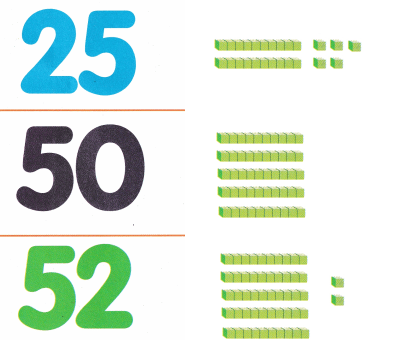Math Talk
Mathematical Processes
How did you figure out how many tens and ones are in 52?
Explanation:
1 line = 10 blocks
5 lines = 50
1 dot = 1 block
2 dots = 2
50 + 2 = 52

Model and Draw

10 tens is the same as 1 hundred.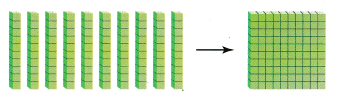Finish the quick picture to show 120.Explanation:
1 box = 100
1 line = 10 blocks
2 lines = 20
1 dot = 1 block
0 dots = 0
100 + 20 + 0 = 120

Share and Show

Use your MathBoard andto show hundreds, tens, and ones. Write the numbers.

Question 1.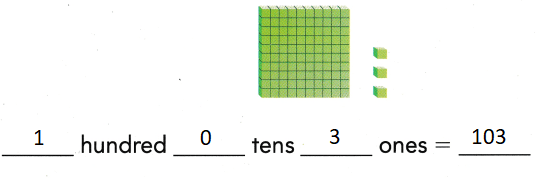Explanation:
Adding of hundreds tens and ones by
base ten blocks method
100 + 30 + 0= 130

Question 2.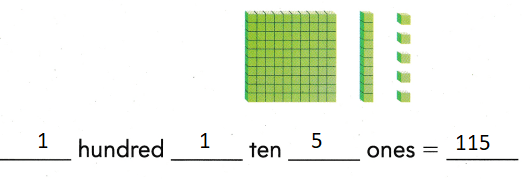Explanation:
Adding of hundreds tens and ones by
base ten blocks method
100 + 10 + 5 = 115

Problem Solving

Write the numbers.

Question 3.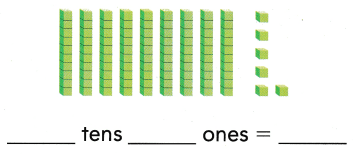Explanation:
Adding of hundreds tens and ones by
base ten blocks method
90 + 6 = 96

Question 4.Explanation:
Adding of hundreds tens and ones by
base ten blocks method
80 + 4 = 84

Draw a quick picture to show the number. Write how many hundreds, tens, and ones there are.

Question 5.
H.O.T. Moe has a group of 70 red feathers and 30 brown feathers.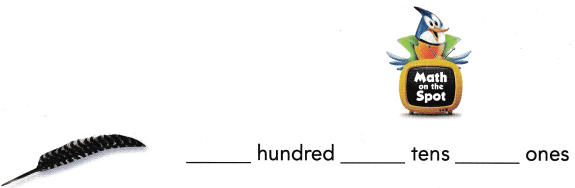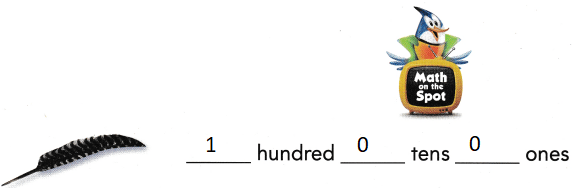Explanation:
Moe has a group of 70 red feathers and 30 brown feathers.
70 +30 = 100

Question 6.
H.O.T. Multi-Step Halley has 40 blocks. Daniel has 50 blocks. Molly has 2 groups of 10 blocks.
____ hundred ___ ten ___ ones
1 hundred 1 ten 0 ones
Explanation:
Halley has 40 blocks.
Daniel has 50 blocks.
Molly has 2 groups of 10 blocks.
40 + 50 + 10 + 10  = 110

Question 7.
A squirrel is getting ready for winter. He has hidden 98 acorns. Which shows this number?
(A) 1 hundred 9 tens 8 ones
(B) 8 tens 9 ones
(C) 9 tens 8 ones
Explanation:
98 = 9 tens and 8 ones
90 + 8 = 98

Question 8.
A dog digs 10 holes. She hides 10 bones in each hole. She has 9 bones left to hide. Which number is 10 tens and 9 more?
(A) 19
(B) 91
(C) 109
Explanation:
10 tens = 100
9 ones = 9
100 + 9 = 109

Question 9.
Use Tools Toni laid down 1 hundred. Then she laid down 1 ten and 5 ones. What number does her model show?(A) 105
(B) 115
(C) 15
Explanation:
100 + 10 + 5 = 115
115 is the number that the model show

Question 10.
Texas Test Prep Which number does the model show?(A) 96
(B) 69
(C) 95
Explanation:
90 + 6 = 96
9 tens and 6 ones

Take Home Activity

• Give your child numbers from 50 to 100. Ask your child to draw a picture to show the tens and the ones in each number, and then write the number.

### Texas Go Math Grade 1 Lesson 1.5 Homework and Practice Answer Key

Use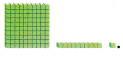Write the numbers.

Question 1.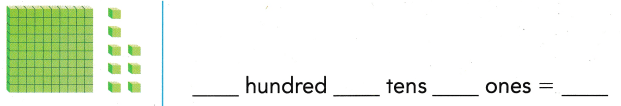Explanation:
Adding of hundreds tens and ones by
base ten blocks method
100 + 0 + 8 = 108

Question 2.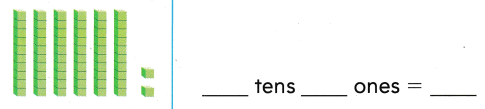Explanation:
Adding of hundreds tens and ones by
base ten blocks method
60 + 2 = 62

Question 3.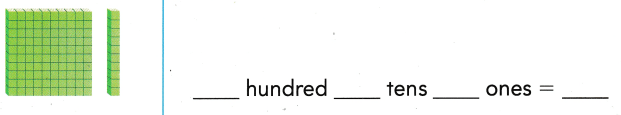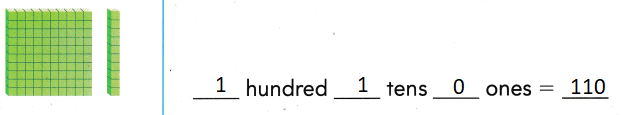Explanation:
Adding of hundreds tens and ones by
base ten blocks method
100 + 10 + 0 = 110

Problem Solving

Draw a quick picture. Write the numbers.

Question 4.
Multi-Step Ali has 10 red buttons, 60 green buttons, and 5 blue buttons.
___ hundred __ tens ___ onesExplanation:
100 + 60 + 5 = 165
1 hundred 6 tens 5 ones

Texas Test Prep’

Lesson Check

Question 5.
Alano collects stickers. She has 102 stickers. Which shows this number?(A) 1 hundred 0 tens 2 ones
(B) 1 hundred 2 tens 0 ones
(C) 1 hundred 1 ten 1 one
Explanation:
100 + 0 + 2 = 102 shows the number
102 shows the number = 1 hundred 0 tens 2 ones

Question 6.
Which number does the model show?(A) 129
(B) 114
(C) 119
Explanation:
100 + 10 + 9 = 119
the model shows 119

Question 7.
Which model shows the number 56?